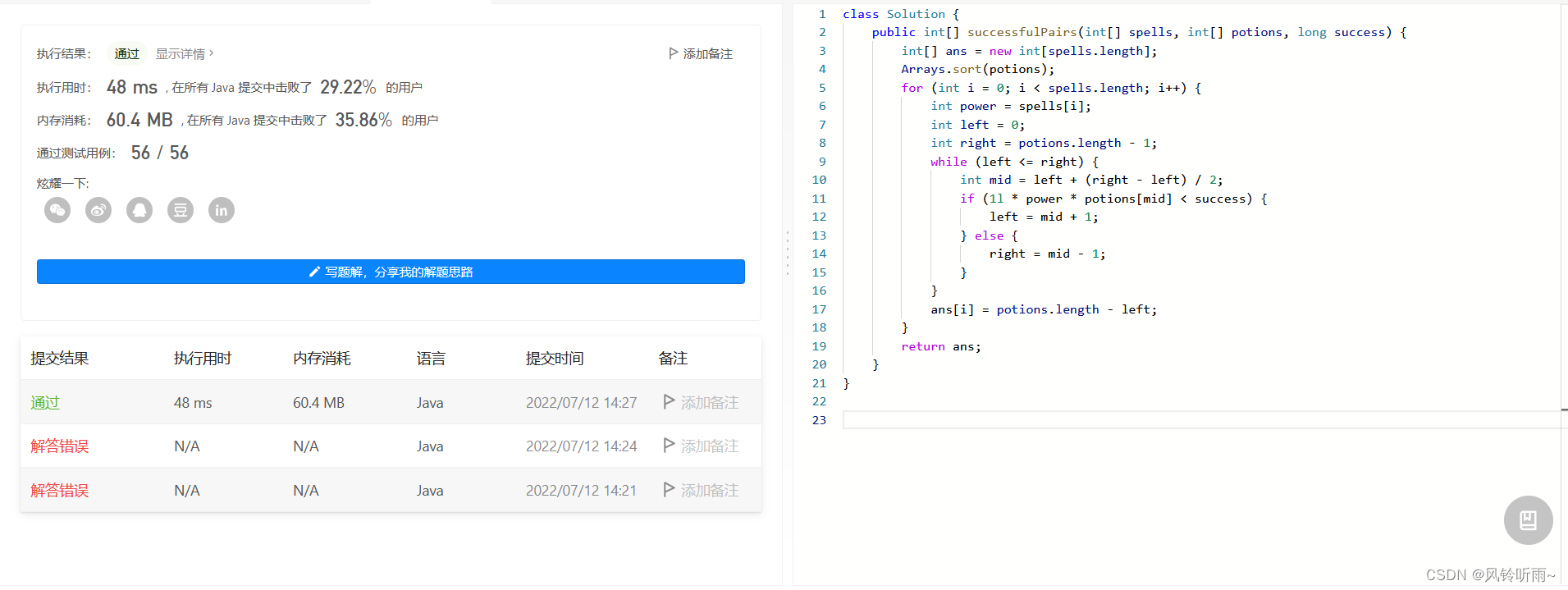> 文档中心 > 【算法刷题】—7.12二分查找应用，数组处理

# 【算法刷题】—7.12二分查找应用，数组处理

• 🧛‍♂️个人主页：杯咖啡
• 💡进步是今天的活动，明天的保证！
• ✨目前正在学习：SSM框架,算法刷题
• 🙌牛客网，刷算法过面试的神级网站，用牛客你也牛。 👉免费注册和我一起学习刷题👈
• 🐳希望大家多多支持🥰一起进步呀！
• 😎Love is the one thing we’are capable of perceiving that transcends time and space.
爱是我们唯一能够感知的可以超越时空维度的事物。-《星际穿越》

# ✨今日算法三题

1.特殊数组的特征值
2.在D天内送达包裹的能力
3.咒语和药水的成功对数

### 文章目录

• ✨今日算法三题
• 1.特殊数组的特征值
• 题目描述
• 思路详解
• 代码与结果
• 2.在D天内送达包裹的能力
• 题目描述
• 思路详解
• 代码与结果
• 3.咒语和药水的成功对数
• 题目描述
• 思路详解
• 代码与结果
• ✨总结

# 1.特殊数组的特征值

## 题目描述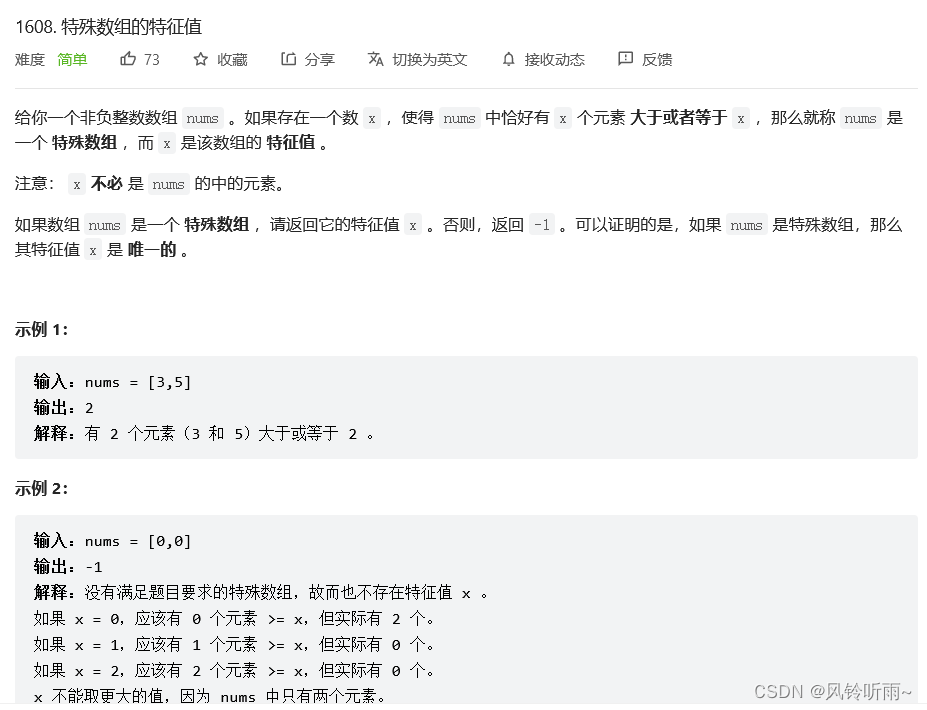## 代码与结果

class Solution {    public int specialArray(int[] nums) { Arrays.sort(nums); int n = nums.length; int left = 0, right = n; while (left <= right) {     int x = (left + right) >> 1;     int idx = binarySearch(nums, x); // nums中第一个大于等于x的元素位置     if (x == n - idx) {  return x;     } else if (x < n - idx) { // 大于等于x的元素太多了，所以下一轮搜索要增大x的取值范围  left = x + 1;     } else { // 反之，减少x的取值范围  right = x - 1;     } } return -1;    } private static int binarySearch(int[] nums, int x) { int left = 0, right = nums.length - 1; while (left <= right) {     int mid = (left + right) >> 1;     int val = nums[mid];     if (val >= x) {  right = mid - 1;     } else {  left = mid + 1;     } } return left;    }}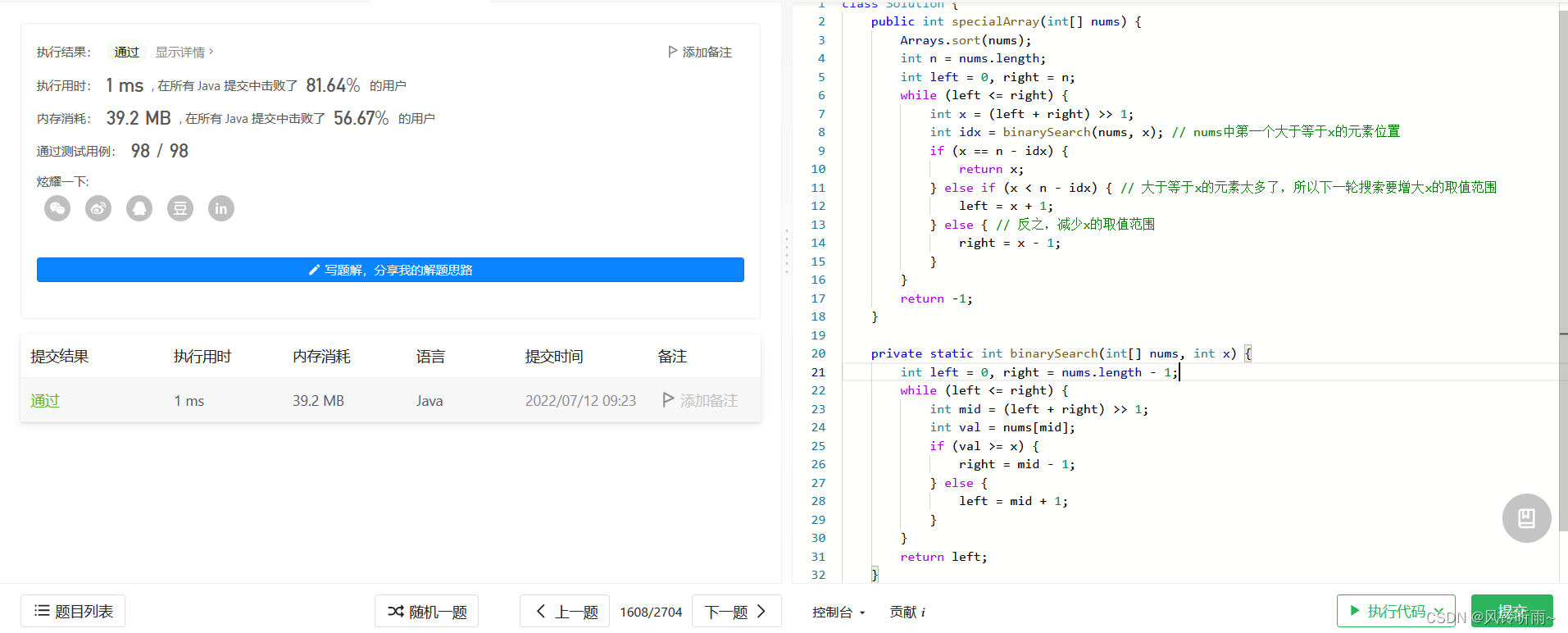# 2.在D天内送达包裹的能力

## 题目描述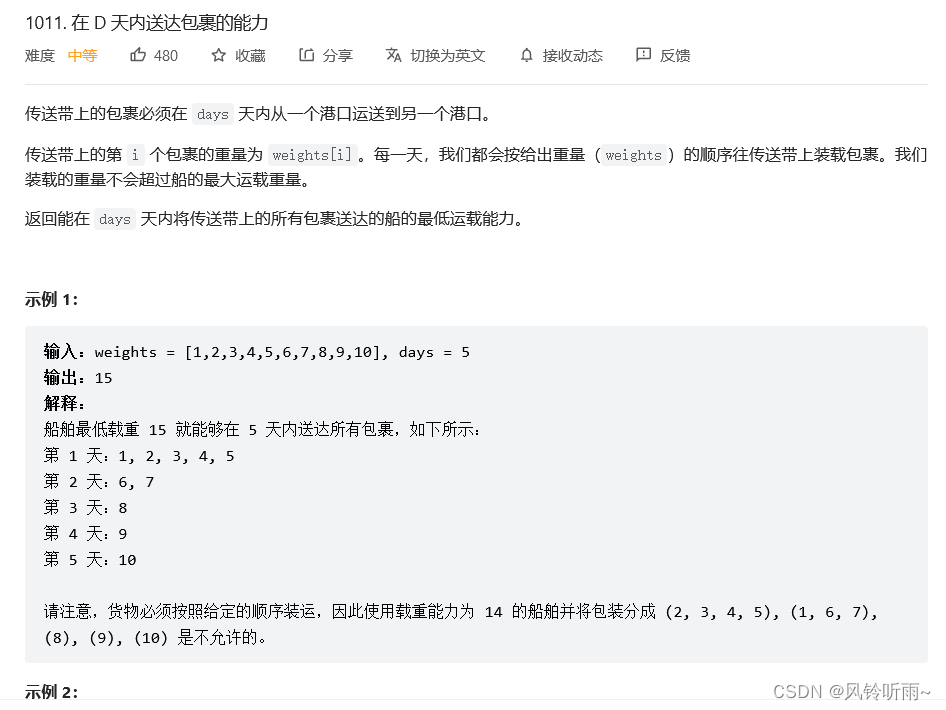## 代码与结果

class Solution {    public int shipWithinDays(int[] weights, int days) { // 确定二分查找左右边界 int left = Arrays.stream(weights).max().getAsInt(), right = Arrays.stream(weights).sum(); while (left < right) {     int mid = (left + right) / 2;     // need 为需要运送的天数     // cur 为当前这一天已经运送的包裹重量之和     int need = 1, cur = 0;     for (int weight : weights) {  if (cur + weight > mid) {      ++need;      cur = 0;  }  cur += weight;     }     if (need <= days) {  right = mid;     } else {  left = mid + 1;     } } return left;    }}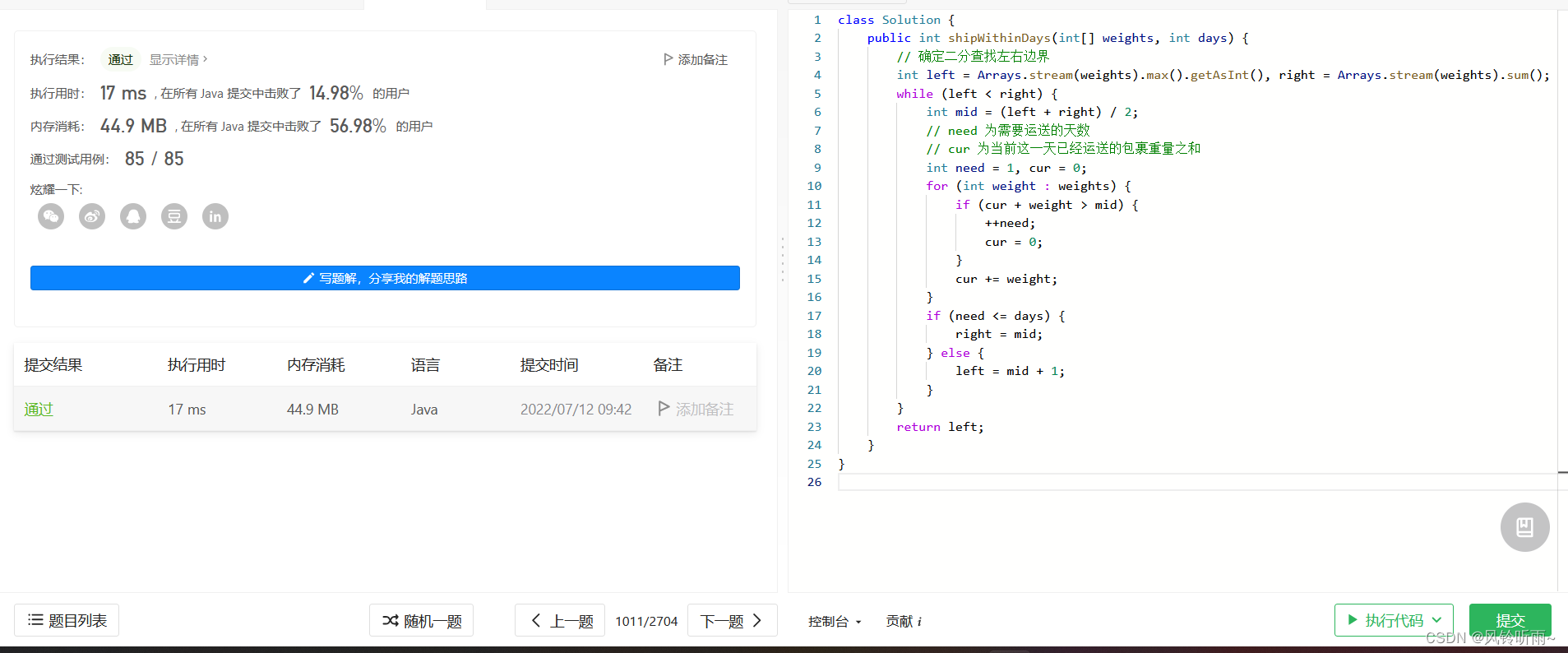# 3.咒语和药水的成功对数

## 题目描述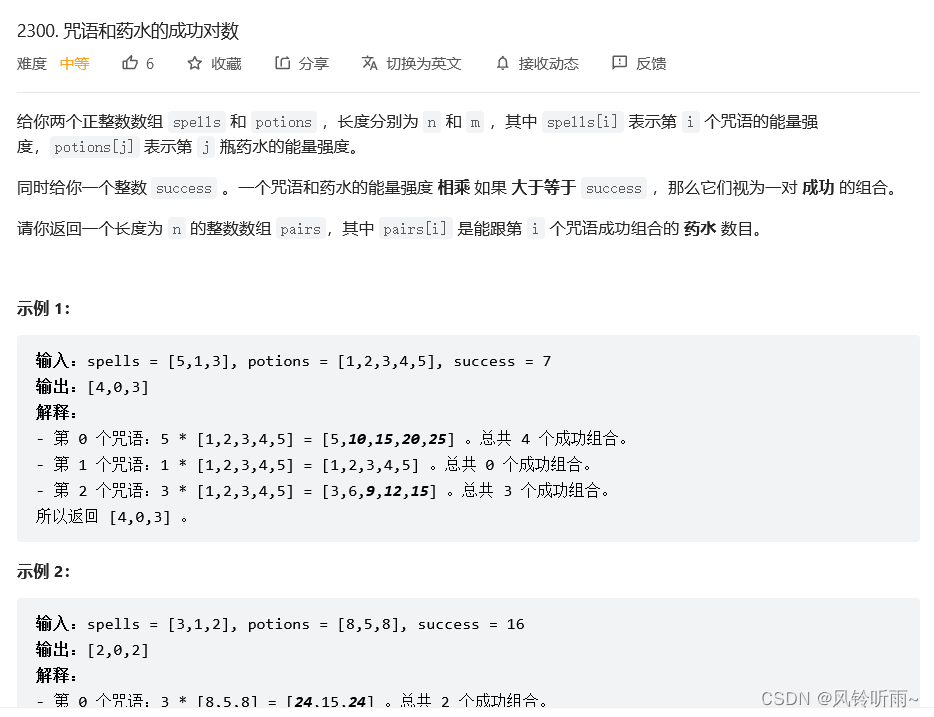## 代码与结果

class Solution {    public int[] successfulPairs(int[] spells, int[] potions, long success) { int[] ans = new int[spells.length]; Arrays.sort(potions); for (int i = 0; i < spells.length; i++) {     int power = spells[i];     int left = 0;     int right = potions.length - 1;     while (left <= right) {  int mid = left + (right - left) / 2;  if (1l * power * potions[mid] < success) {      left = mid + 1;  } else {      right = mid - 1;  }     }     ans[i] = potions.length - left; } return ans;    }}